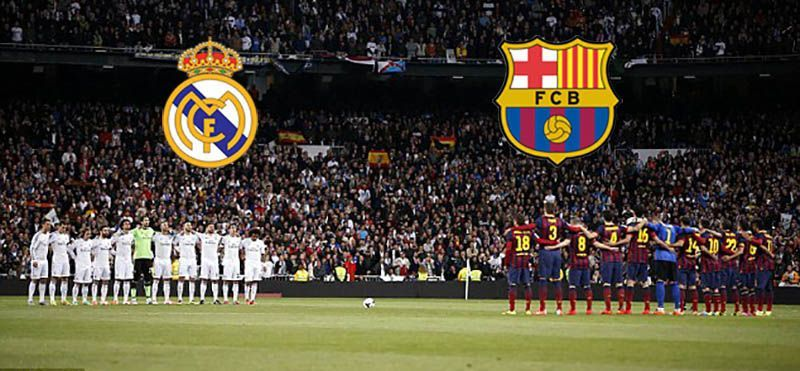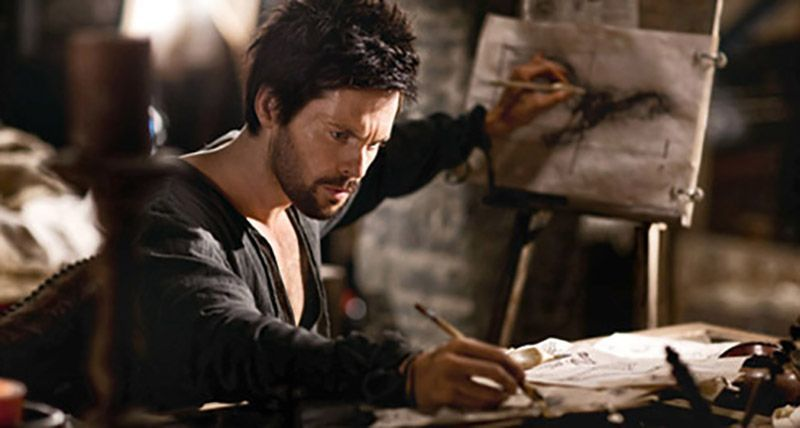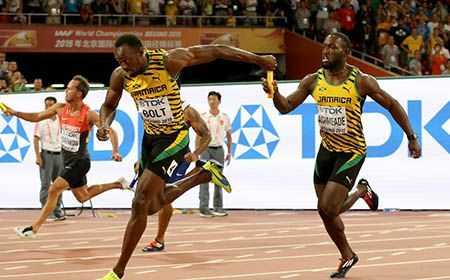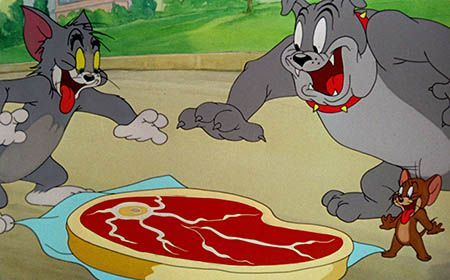## Free Samples# Pictograph

Representing your world in pictures! See how the type and number of a quantity can be mathematically represented pictorially.# Venn Diagram

The easiest way to imagine sets visually. See the power of Venn Diagrams.# Venn Diagram Example

Learn how to use Venn Diagrams to solve real world problems with these interesting examples.# Sequences

Learn about simple sequences with an interesting example of a relay race.# Sequences Example

Learn about sequences with these interesting solved examples.# Factors

Knowledge of factors can win you the world cup! Learn about factors with this interesting example.# Introduction to Fractions

Learn fractions with Tom and Jerry. Understand fractions as equal parts of a whole.